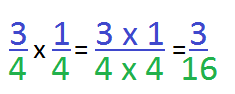# Multiplying Fractions with Like Denominators

Instructor: Christine Quist

Christine has taught 4th-5th grade, has worked as a Paraprofessional for Adult Learners, and has a master's degree in Curriculum and Instruction for Elementary Education.

Fractions are all around us, and, just like whole numbers, they can be added, subtracted, divided and multiplied. Believe it or not, multiplying fractions is the easiest one of all, and in this lesson, you will learn just how easy it is!

## Fraction Action

You have 1/2 of a cookie and now your mom wants you to share your 1/2 equally with your brother. So, you have to give 1/2 of your 1/2 to your brother. How much of the cookie are you and your brother going to get? To find out, you will need to multiply fractions. Before we get there, let's do a quick review. As you may recall, fractions are parts of a whole. They consist of a numerator, number on top, and a denominator, number on bottom.

When you learned to add and subtract fractions, you learned that you must first have like denominators, meaning the denominators are the same number, and the denominators stayed the same when you were adding or subtracting the fractions. However, when we multiply fractions with like denominators, that is no longer the rule. Instead, we will get a totally different denominator! Let's see how that works.

## Three Easy Steps

Multiplying fractions is as easy as 1, 2, 3!

Step 1: Multiply the numerators

Step 2: Multiply the denominators

Step 3: Simplify the end result

To simplify means to make the fraction into the smallest numbers possible by finding the largest number that can divide both the numerator and denominator. For example, 2/4 can be simplified to 1/2 because both the numerator and denominator can be divided by 2. So 2/4 ÷ 2/2 = 1/2. We use 1/2 because it is easier to see and understand. Let's put our three steps to the test.

### Example #1

Let's multiply 3/4 x 1/4 following our 'Three Easy Steps:'

Step 1: Multiply the numerators

3/4 x 1/4 = 3 x 1 = 3

Step 2: Multiply the denominators

3/4 x 1/4 = 4 x 4 = 16

So our answer to 3/4 x 1/4 = 3/16. Don't forget Step 3.

Step 3: Simplify

Is there any number besides 1 that can go into both 3 and 16? There is not. So, 3/16 is as simplified as it can get!### Example #2

Let's really test your skills! Let's multiply 3/10 x 5/10. Don't forget your steps.

Step 1: Multiply the numerators

3/10 x 5/10 = 3 x 5 = 15

Step 2: Multiply the denominators

3/10 x 5/10 = 10 x 10 = 100

Step 3: Simplify

To unlock this lesson you must be a Study.com Member.

### Register to view this lesson

Are you a student or a teacher?

#### See for yourself why 30 million people use Study.com

##### Become a Study.com member and start learning now.
Back
What teachers are saying about Study.com

### Earning College Credit

Did you know… We have over 200 college courses that prepare you to earn credit by exam that is accepted by over 1,500 colleges and universities. You can test out of the first two years of college and save thousands off your degree. Anyone can earn credit-by-exam regardless of age or education level.